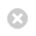Close

UCL Quantum Science and Technology Institute

Home# How we stopped worrying about scaling and started learning about quantum states

29 March 2019

For the first time, researchers at UCL have experimentally demonstrated that even for complex quantum systems it is possible to perform certain learning tasks using only a linear number of measurements.UNIVERSITY COLLEGE LONDON

PhD student Rocchetto and colleagues reported today on Science Advances about the first experimental demonstration that quantum states can be learned using only a linear number of measurements. The experiments were performed on a photonic system with up to 6 qubits and were conducted in collaboration with Fabio Sciarrino’s lab at Università Sapienza in Rome.

As the complexity of quantum systems increases exponentially with system size (what researchers call “exponential scaling”) our ability to fully characterise them is limited to no more than a few qubits. In 2007 Scott Aaronson, co-author and Professor of Computer Science at the University of Texas at Austin, theoretically proved that in a probabilistic setting, this complexity is only apparent and quantum states can be approximately learned using only a linear number of measurements.

This result has interesting applications for our ability to model physical systems. An important element of every physical model is a set of equations that describe the interdependent behaviour of the variables of the system (e.g. time, space, energy). Very few models in physics can be solved analytically, i.e. with pen and paper calculations, without using simplifying assumptions. In the case of quantum mechanics this problem is particularly critical as even for very small systems (less than 50 particles), direct numerical calculations become intractable even for the fastest supercomputers.

If we cannot find theoretically motivated assumptions to reduce the complexity of our models it is conceivable to use machine learning methods to simplify the problem by using patterns in data. A popular machine learning tool is deep learning (a type of algorithm based on artificial neural networks) but with this model it is difficult to formulate precise statements on when the predictions are no longer valid, an important requirement in physics. In the case of Aaronson’s theorem, it is instead possible to quantify precisely the generalisation performance of the model.

“Our results highlight the power of computational learning theory to investigate quantum information - explains Rocchetto - providing the ﬁrst experimental demonstration that quantum states can be “probably approximately learned” with access to a number of copies of the state that scales linearly with the number of qubits, and pave the way to probing quantum states at new, larger scales - and he adds - "As a theorist, it is fascinating to see how formal models of learning can help us to gain novel insights on the nature of quantum states.

The researchers’ work introduced to the wider physics community a formal model of learning that enables the development of mathematically precise statements on the statistical and computational hardness of a learning problem. This type of model could have many applications in physics where the aim is to quantify the quality of the predictions.

“Machine learning holds great promise for the advancement of experimental quantum information but it is often unclear to what extent we can trust the outcomes of a learning algorithm - remarks co-author Fabio Sciarrino - and adds: “The techniques we implemented have provable guarantees that enormously facilitate the experimental analysis."

The team hopes that in the future, these tools could help to tackle hard analytical problems for which no analytical or numerical solution is available and at the same time provide insights into the underlying physical properties of the larger systems in order to study the many-particle entangled states that arise in fields such as chemistry, condensed-matter physics and quantum information.

IMAGE CAPTION: Close up of the experimental apparatus used for producing and measuring the quantum states. The crystal visible at the centre of the figure is where the generation of the qubits occurs. Credit: Gonzalo Carvacho.

MEDIA CONTACT: Agnese Abrusci, UCL Quantum Science and Technology Institute